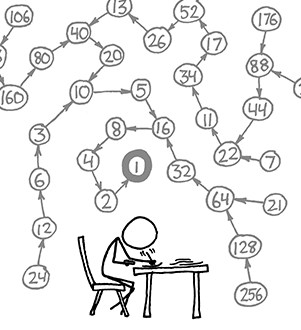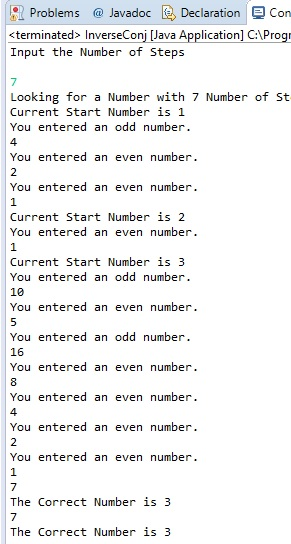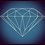# Inverse Collatz ConjectureSchools out now, and for my final grade my programming teacher gave us the option to come up with our own project to research about, problem solve, code, and present to the class. My project dealt with the Collatz conjecture just because some friends of mine who i visited last year during the AOTM summer camp told me about it, and got me extremely interested in how it works.

For people who don't know what the Collatz conjecture is; basically all it states is that if you have a even number plug that number into this equation: n/2, and if you have an odd number use this equation: 3n+1. The Conjecture states that no matter what number you put in, you will eventually end up at the number 1.

For example, the number 3:

3 is odd so we use 3n+1

3(3)+1 = 10

10 is even so we use n/2

10/2 = 5 3(5)+ 1 = 16 16/2 = 8 8/2 = 4 4/2 = 2 2/2 = 1

Given these standards, we can see that each number has a given number of "steps", or number of times you have to use the conjecture to reach the number 1. So like for the number 3 we got 3, 10, 5, 16, 8, 4, 2 , and 1 which is 8 steps(7 if 3 is not included). Here is where my final project comes into play. I asked myself the question "If given the number of steps, can we find the number or numbers that have that amount of steps? In other words, if i was to say "what number has 8 steps?" how could i find that number, and whether or not there are multiple numbers that have that many steps.

So basically my question is can we find all real numbers that derive from the steps of any real number using the Collatz conjecture.

To do this a friend of mine and I came together and wrote this code in java that basically inputs a number starting from 1 into the Collatz conjecture. Then it counts the number of steps it takes for that number, then checks to see if the number of steps matches the number inputted by the user. If the number of steps does not match the number asked then it tries the next number and so on.

The written code can be found here

Class here

This code works and all and i got a 100 on my final grade for it, but it doesn't answer the question of finding all integers. Instead, the program just finds the first number and sends it to the user.TL;DR How can I update my code so that it lists ALL of the numbers that have the given number of steps?Note by Nathan Antwi
6 years, 1 month ago

This discussion board is a place to discuss our Daily Challenges and the math and science related to those challenges. Explanations are more than just a solution — they should explain the steps and thinking strategies that you used to obtain the solution. Comments should further the discussion of math and science.

When posting on Brilliant:

• Use the emojis to react to an explanation, whether you're congratulating a job well done , or just really confused .
• Ask specific questions about the challenge or the steps in somebody's explanation. Well-posed questions can add a lot to the discussion, but posting "I don't understand!" doesn't help anyone.
• Try to contribute something new to the discussion, whether it is an extension, generalization or other idea related to the challenge.

MarkdownAppears as
*italics* or _italics_ italics
**bold** or __bold__ bold
- bulleted- list
• bulleted
• list
1. numbered2. list
1. numbered
2. list
Note: you must add a full line of space before and after lists for them to show up correctly
paragraph 1paragraph 2

paragraph 1

paragraph 2

[example link](https://brilliant.org)example link
> This is a quote
This is a quote
    # I indented these lines
# 4 spaces, and now they show
# up as a code block.

print "hello world"
# I indented these lines
# 4 spaces, and now they show
# up as a code block.

print "hello world"
MathAppears as
Remember to wrap math in $$ ... $$ or $ ... $ to ensure proper formatting.
2 \times 3 $2 \times 3$
2^{34} $2^{34}$
a_{i-1} $a_{i-1}$
\frac{2}{3} $\frac{2}{3}$
\sqrt{2} $\sqrt{2}$
\sum_{i=1}^3 $\sum_{i=1}^3$
\sin \theta $\sin \theta$
\boxed{123} $\boxed{123}$

Sort by:

Wrote this up in python real quick but not super experience with code so it's not necessarily super efficient:

steps=int(raw_input("Enter integer number of steps: "))
arr=
next_arr=[]
prev_num=[]
for i in range(0, steps-1):
for x in arr:
if(x%3==1 and x%2==0):
next_arr.append((x-1)/3)
next_arr.append(2*x)
for x in next_arr:
if x in prev_num:
next_arr.remove(x)
prev_num.extend(arr)
arr=next_arr[:]
next_arr=[]
print arr


Takes initial array "arr" of values from last collatz cycle, at the beginning this is just 1. Then it stores another array of potential values for the next level of the collatz cycle. Finally there is a store of previous numbers so you don't repeat old numbers that say, had a collatz number of 4 showing up in level 7. In the for loop, it checks to see, for each number in the current level, what numbers could get the number from the next level up and checks to see that they are each valid. Then it adds the array to the previous numbers and makes the current array the next array and just loops for the number of steps you enter.

For example entering 8 at the prompt returns [3, 20, 21, 128]

- 6 years, 1 month ago

Holy crap. This is very nice. Props and #Respect. Good job hahaha.

- 6 years, 1 month ago

Congratulations on getting a 100.

The Collatz conjecture is for integers, not real numbers

- 6 years, 1 month ago

Positive integer, to be precise.

- 6 years, 1 month ago

Ahhh my mistake.. Thanks

- 6 years, 1 month ago

You can even try this out for fun.

- 6 years, 1 month ago